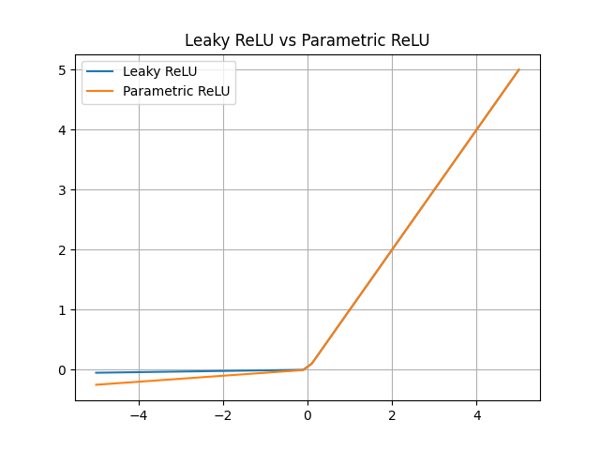Trusted answers to developer questions
Trusted Answers to Developer Questions

Related Tags

relu
parametric relu

# What is Parametric ReLU?Muhammad Nabeel

Grokking Modern System Design Interview for Engineers & Managers

Ace your System Design Interview and take your career to the next level. Learn to handle the design of applications like Netflix, Quora, Facebook, Uber, and many more in a 45-min interview. Learn the RESHADED framework for architecting web-scale applications by determining requirements, constraints, and assumptions before diving into a step-by-step design process.

Rectified Linear Unit (ReLU) is an activation function in neural networks. It is a popular choice among developers and researchers because it tackles the vanishing gradient problemThe gradients of activation functions, like sigmoid, become very small. This makes it difficult to train bigger models.. A problem with ReLU is that it returns zero for any negative value input. So, if a neuron provides negative input, it gets stuck and always outputs zero. Such a neuron is considered dead. Therefore, using ReLU may lead to a significant portion of the neural network doing nothing.

Researchers have proposed multiple solutions to this problem. Some of them are mentioned below:

• Leaky ReLU
• Parametric ReLU
• ELU
• SELU

In this Answer, we discuss Parametric ReLU.

### Parametric ReLU

The mathematical representation of Parametric ReLU is as follows:

Here, $y_i$is the input from the $i\text{th}$ layer input to the activation function. Every layer learns the same slope parameter denoted as$\alpha_i$. In the case of CNN, $i$represents the number of channels. Learning the parameter, $\alpha_i$boosts the model's accuracy without the additional computational overhead.

Note: When $\alpha_i$is equal to zero, the function $f$ behaves like ReLU. Whereas, when $\alpha_i$is equal to a small number (such as 0.01), the function $f$behaves like Leaky ReLU.

The above equation can also be represented as follows:

Using Parametric ReLU does not burden the learning of the neural network. This is because the number of extra parameters to learn is equal to the number of channels. This is relatively small compared to the number of weights the model needs to learn. Parametric ReLU gives a considerable rise in the accuracy of a model, unlike Leaky ReLU.

If the coefficient $\alpha_i$is shared among different channels, we can denote it with a$\alpha$.

### Parametric ReLU vs. Leaky ReLU

In this section, we compare Parametric ReLU with the performance of Leaky ReLU.Leaky ReLU vs Parametric ReLU

Here, we plot Leaky ReLU with $\alpha = 0.01$and have Parametric ReLU with $\alpha = 0.05$. In practice, this parameter is learned by the neural network and changes accordingly.

RELATED TAGS

relu
parametric relu

CONTRIBUTORMuhammad Nabeel